### IMO Shortlist 2006 problem G2

Kvaliteta:
Avg: 0,0
Težina:
Avg: 6,0
Let$ABC$ be a trapezoid with parallel sides$AB > CD$. Points$K$ and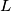$L$ lie on the line segments$AB$ and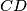$CD$, respectively, so that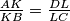$\frac {AK}{KB} = \frac {DL}{LC}$. Suppose that there are points$P$ and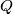$Q$ on the line segment$KL$ satisfying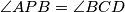$\angle{APB} = \angle{BCD}$ and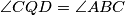$\angle{CQD} = \angle{ABC}$. Prove that the points$P$,$Q$,$B$ and$C$ are concylic.
Izvor: Međunarodna matematička olimpijada, shortlist 2006